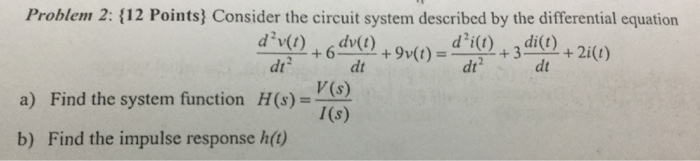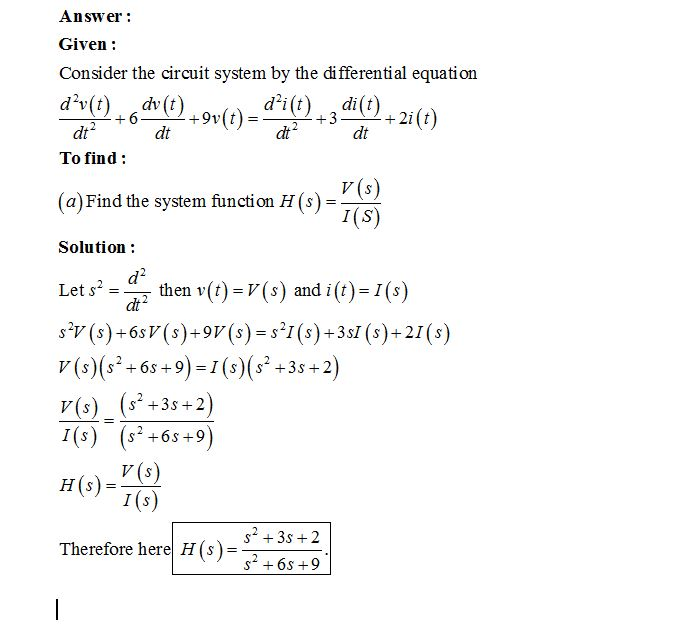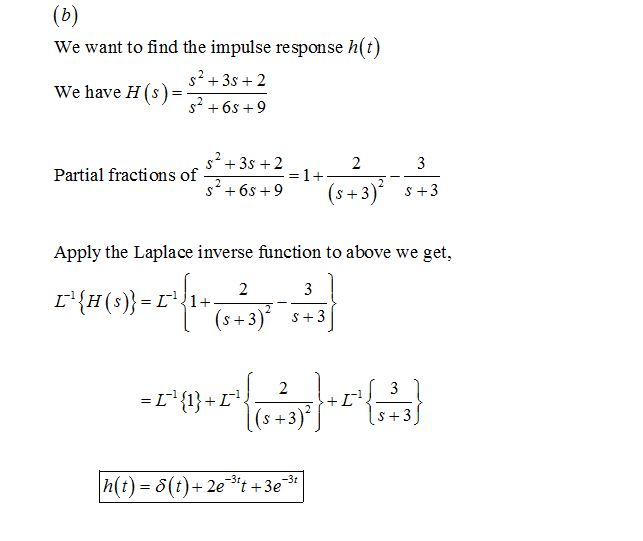# Answered! Consider the circuit system described by the differential equation d^2 v(t)/dt^2 + 6 dv(t)/dt + 9v (t) = d^2 (t)/dt^2…

Consider the circuit system described by the differential equation d^2 v(t)/dt^2 + 6 dv(t)/dt + 9v (t) = d^2 (t)/dt^2 + 3 di(t)/dt + 2i(t) a) Find the system function H(s) = V(s)/I(s) b) Find the impulse response h(t)